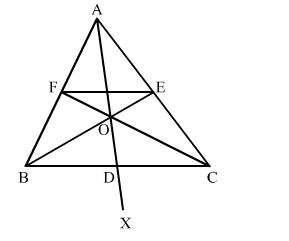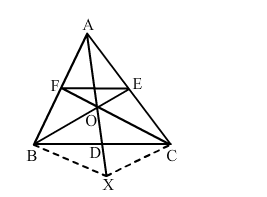# In the given figure, side BC of ∆ABC is bisected at D and O is any point on AD.Question:

In the given figure, side BC of ∆ABC is bisected at D and O is any point on ADBO and CO produced meet AC and AB at E and F, respectively, and AD is produced to X, so that D is the midpoint of OX. Prove that AO : AX = AF : AB and show that EF ∥ BC.Solution:Join BX and CX.
It is given that BC is bisected at D.
BD = DC ​
It is also given that OD = OX
The diagonals OX and BC of quadrilateral BOCX  bisect each other.
Therefore, BOCX is a parallelogram.

$\therefore B O \| C X$ and $B X \| C O$

$B X \| C F$ and $C X \| B E$

$B X \| O F$ and $C X \| O E$

Applying Thales' theorem in $\triangle A B X$, we get:

$\frac{A O}{A X}=\frac{A F}{A B} \quad \ldots(1)$

Also, in $\triangle A C X, C X \| O E$.

Therefore by Thales' theorem, we get:

$\frac{A O}{A X}=\frac{A E}{A C} \quad \cdots(2)$

From (1) and (2), we have:

$\frac{A F}{A B}=\frac{A E}{A C}$

Applying the converse of Thales' theorem in $\triangle A B C, E F \| C B$.

This completes the proof.